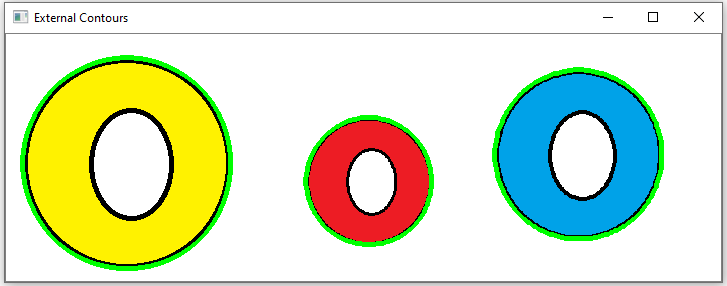﻿ How to Draw All Contours of an Image in Python using OpenCV ﻿# How to Draw All Contours of an Image in Python using OpenCVIn this article, we show how to draw all contours of an image in Python using the OpenCV module.

Finding contours of an image is very important in computer vision, because we need to find various objects. We find various objects by their contours.

OpenCV has a cv2.drawContours() function, which allows us to draw the contours of an image.

Now there are really 2 distinctions for contours of objects in images.

There are external contours- the outermost contour of an object in the image.

There are internal contours- contours within an object in the image.

It's important to make a distinction, as we can draw only external contours or we can draw the external and internal contours of an image.

So in this example, we're going to try a simple image shown below with donuts.When we use the OpenCV cv2.drawContours() to draw the external contours of this image, we get the following image shown below.You can see that OpenCV outlines the external contours, or outermost layers, of the 3 donuts (or Os) found in the image.

So, in OpenCV, we can outline the outer, or external, contours of an image.

However, if we want to, we can outline all contours of an object in an image, including the external contours and internal contours.

If we draw all contours of the original image with the 3 donuts, we get the following image shown below.You now see that now the external and internal contours of objects in the image are outlined.

So let's now get into the code of how to draw contours of an image.

### Drawing External Contours of an Image

We will first start with how to draw external contours within an image in Python using OpenCV.

The code is shown below.

Let's now go over this code.

First, we import OpenCV using the line, import cv2

Next, we read in the image that we draw contours of. In this case, it is, Donuts.png

Next, we create a variable, gray, which hold the grayscale version of the original image. This is to simplify the image

We then produce the Canny edges of the image using the cv2.Canny() function. Though this is an optiponal step, this can help produce a better image for the cv2.drawContours() function.

We then create 2 variables, contours and hierarchhy, which find the contours of the image, edges. The second parameter is the cv2.RETR_EXTERNAL, which finds the external contours of the image. The cv2.CHAIN_APPROX_NONE simply stores all possible points of the contours.

We then use the cv2.drawContours() function to draw the external contours of the image. We do this on the original image, image. The second parameter is the contours we want in the image specified in the variable, contours. The third parameter is -1, which specifies that we want all contours to be drawn. If you specified 1, it would simply draw first contour. If you specified 2, it would draw the first 2 contours. -1 draws all contours. The fourth parameter is the color that you want the contour to be shown in. In this example, (0,255,0) represents the color green. The fifth and last parameter is the thickness of the line, in this case, 3. You can vary this as you see fit.

We then show the image with the contours outlined using the cv2.imshow() function.

### Drawing All Contours of an Image

We now draw all contours (external and internal) within an image in Python using OpenCV.

The code is shown below.

So the code is just as before, except for the second parameter of the cv2.findContours() function, we specify, cv2.RETR_LIST. This find all contours, external and internal, of the image.

So we are able to get all contours of an image now.

And this is how we can draw contours of an image in Python using OpenCV.

Related Resources